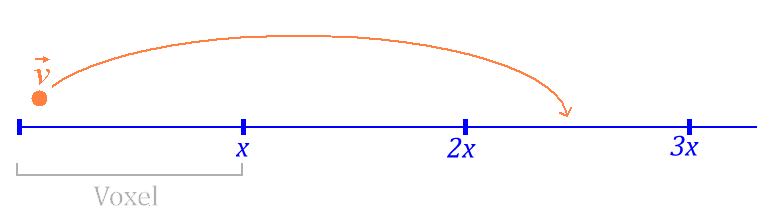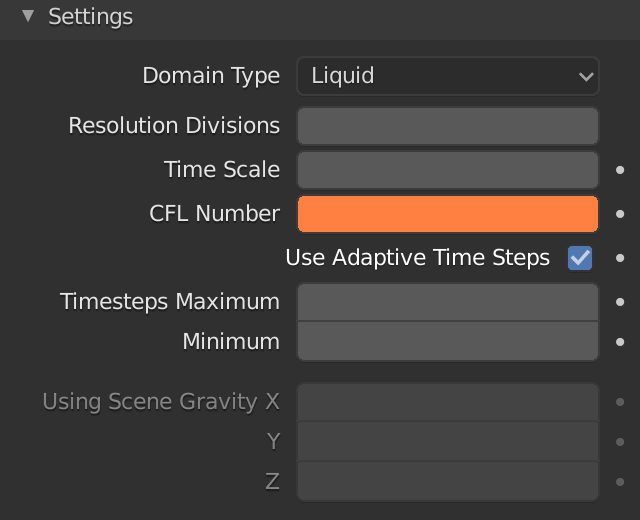# CFL Number

– As you probably know the (differential) equations describing the motion of all the particles are resolved by using numerical methods.
– Honestly I don’t know wich algorithm Blender uses, but I suppose it uses the standard v*(Δt/Δx) for the CFL Number. If you know another formula is used please write me!
– What does the CFL Number represent? Look at this image (voxels are 3-dimensional and this is a one-dimensional example but this reasoning can be extended to a 3D voxel):– If v is too hight, we loose the information that should be stored in the second voxel. The solution is to insert extra Time Steps.
x is the size of a voxel and t the time the particle needs to run it, v=x/t the particle’s velocity.
– If we reduce the velocity, that’s to say v≤x/t, the particle will run one FULL voxel (or LESS).
– If we think in terms of incremental values: v≤Δx/Δt ⇒ v*(Δt/Δx)≤1

CFL≤1 is the condition, for a particle, to travel only from a voxel to a adiacent one.
– Why does the default Blender CFL Number is set to 4.0 instead of 1.0 (or less) ? I think it’s to reduce baking time.

How to choose the right CFL Number for your simulation? Blender is not “real simulations” oriented. Remember we will never obtain a “real” simulation as everything is based on approximate physics that lead to approximate solutions. In general a smaller CFL number means a more ‘realistic’ simulation but if you find your simulation looks better with a greater CFL Number, raise it up!

– In this first example see how different values of CFL Number affects a simulation:

– What about using CFL Numbers greater than one? Again consider again the above image: if for a certain velocity your particle cannot travel ,e.g, 3 voxels, no matter if we rise up the CFL Number, because if your particle cannot travel 3 voxels, will not be able to travel 4 or more, so that no extra Time Steps will be used.

– In this second example see how a simulation remains exactly same from CFL Number = 4.2 up.– Always keep in mind that your simulation is a combination of CFL Number , Timesteps, Resolution Divisions, Video Frame Rate etc..!

Some considerations

– The CFL Number is a necessary (but not sufficient) condition for the convergence of the differential equations that describe the motion of all the particles inside the domain.
– “Convergent Solutions” means that when Δx and Δt (used in the numerical method) tend to zero, this numerical solution will tend to the exact solution.
– Sometimes the CFL Number is confused with a ‘Stability Condition’ (that means that as computation proceeds, errors get smaller). Keep in mind that there are methods stable for arbitrary Δt that, however, are not convergent unless a CLF condition is met.
– Smaller CFL Numbers mean bigger computational times (because of the extra Time Steps).
– CFL Number=0 , make it sense? The CFL Number can be equal to zero only if velocity is equal to zero that’s to say for NO moving fluids or NO time flow. (v*(Δt/Δx)=0 ⇒ v=0 OR Δt=0 ). I think that if you choose CFL NUmber=0 then Blender sets very small value, please let me know if you know the correct answer to this question!